内容摘要

• 图像中的噪声是什么?
• 问题表述
• 机器学习问题提法
• 数据来源
• 探索性数据分析
• 图像去噪的传统滤波器概述
• 用于图像去噪的深度学习模型
• 结果比较
• 未来的工作和改进的范围
• 参考文献

图像中的噪点是什么？

A（x，y）= B（x，y）+ H（x，y）

A（x，y）=噪声图像的函数； B（x，y）=原始图像的函数； H（x，y）=噪声的函数；

机器学习问题提法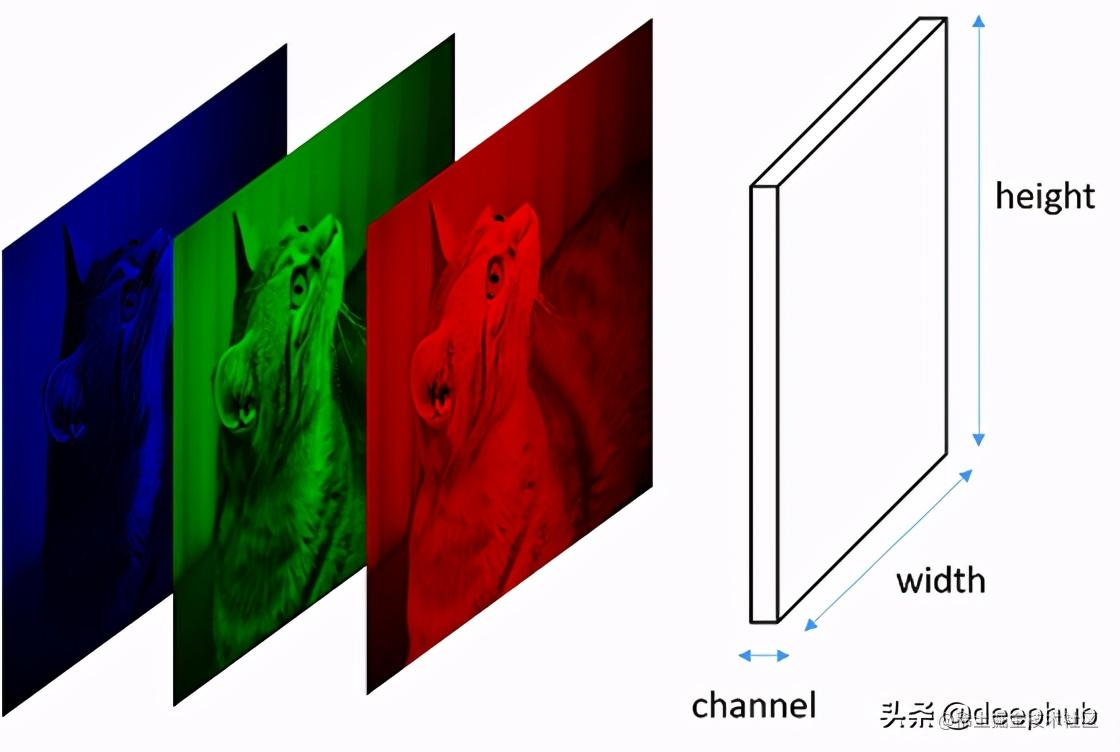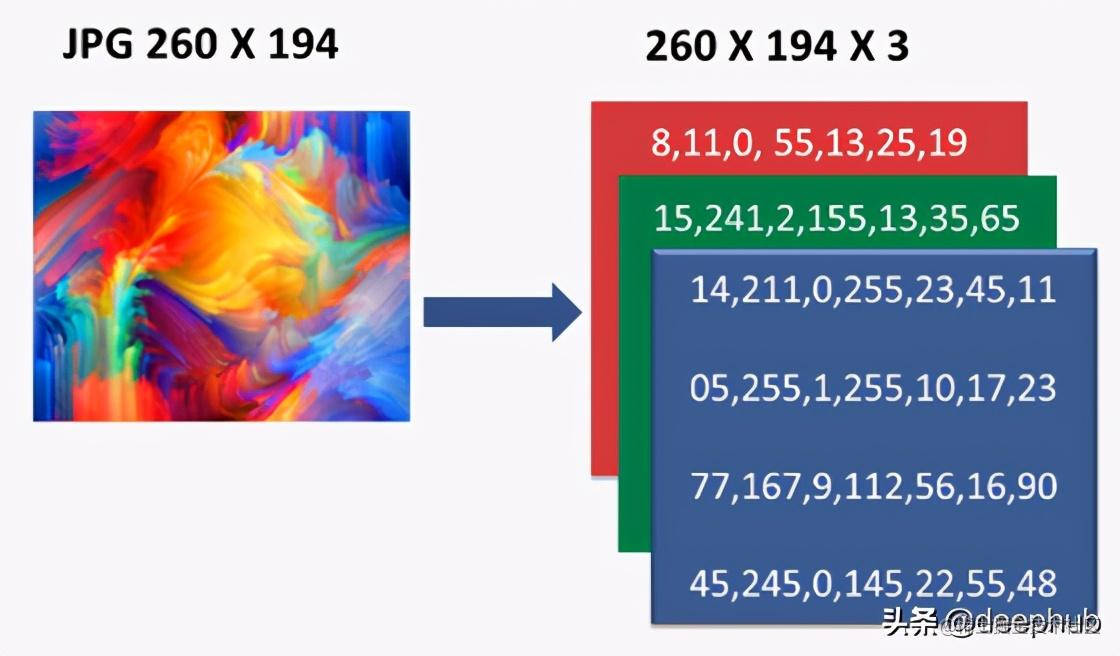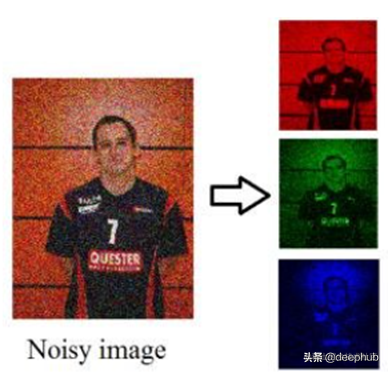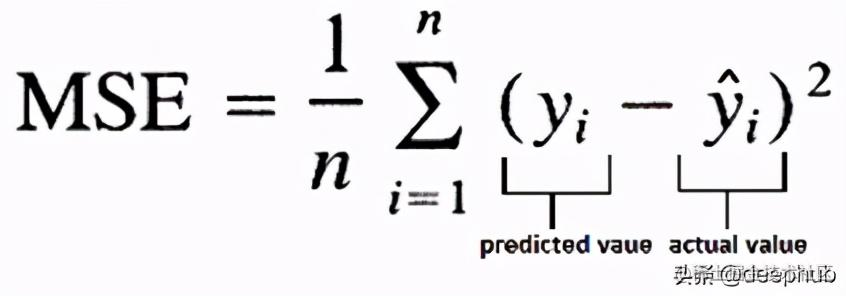• PSNR (Peak Signal to Noise Ratio) 峰值信噪比
• SSIM (Structural Similarity Index Measure) 结构相似性

数据来源

SIDD -包含160对来[噪声-真值]图像

RENOIR -包含80对[嘈杂的-真值]图像

NIND -包含62对[噪声-真值]图像

探索性数据分析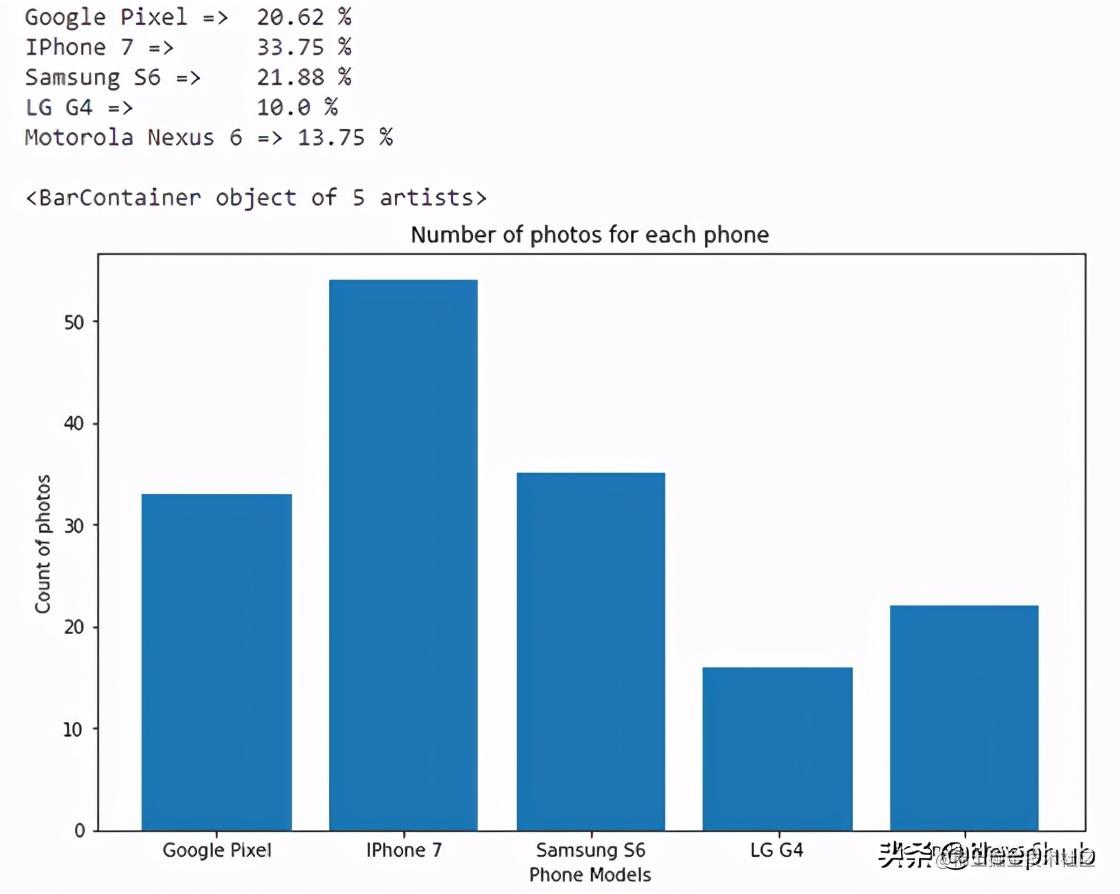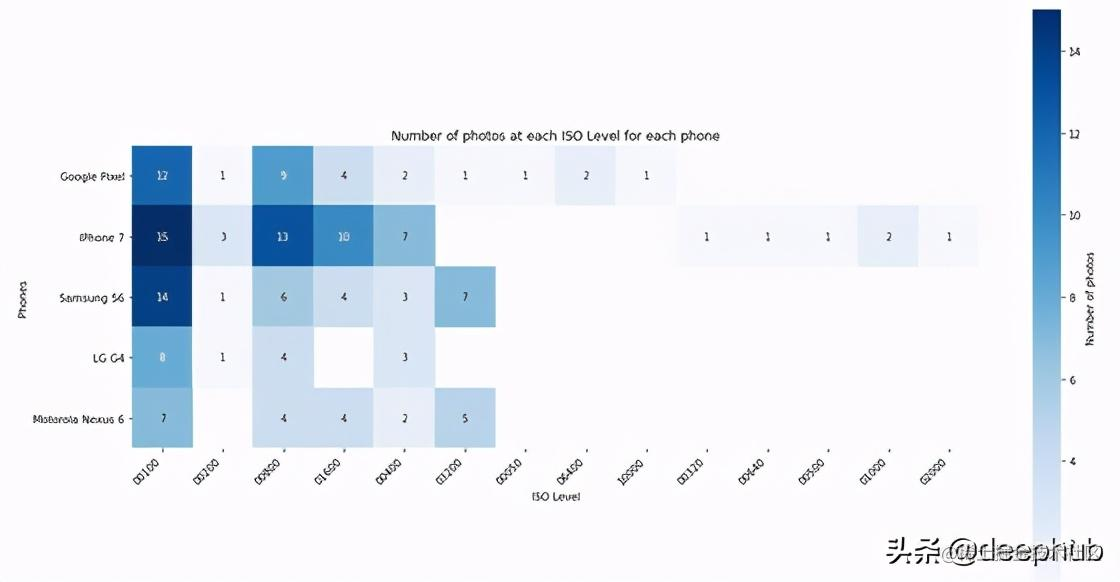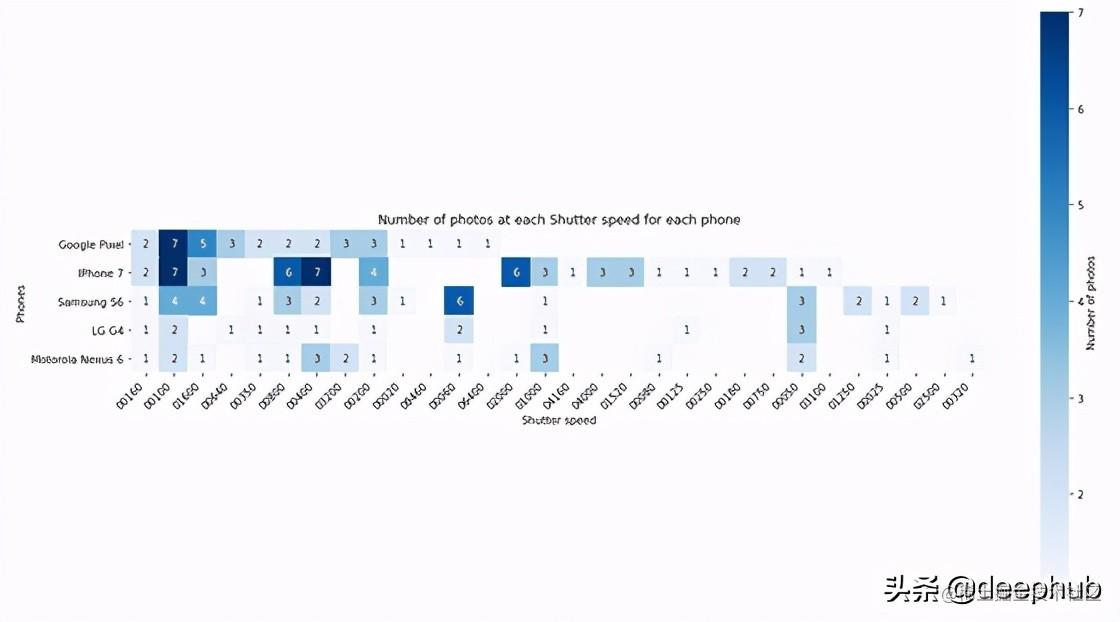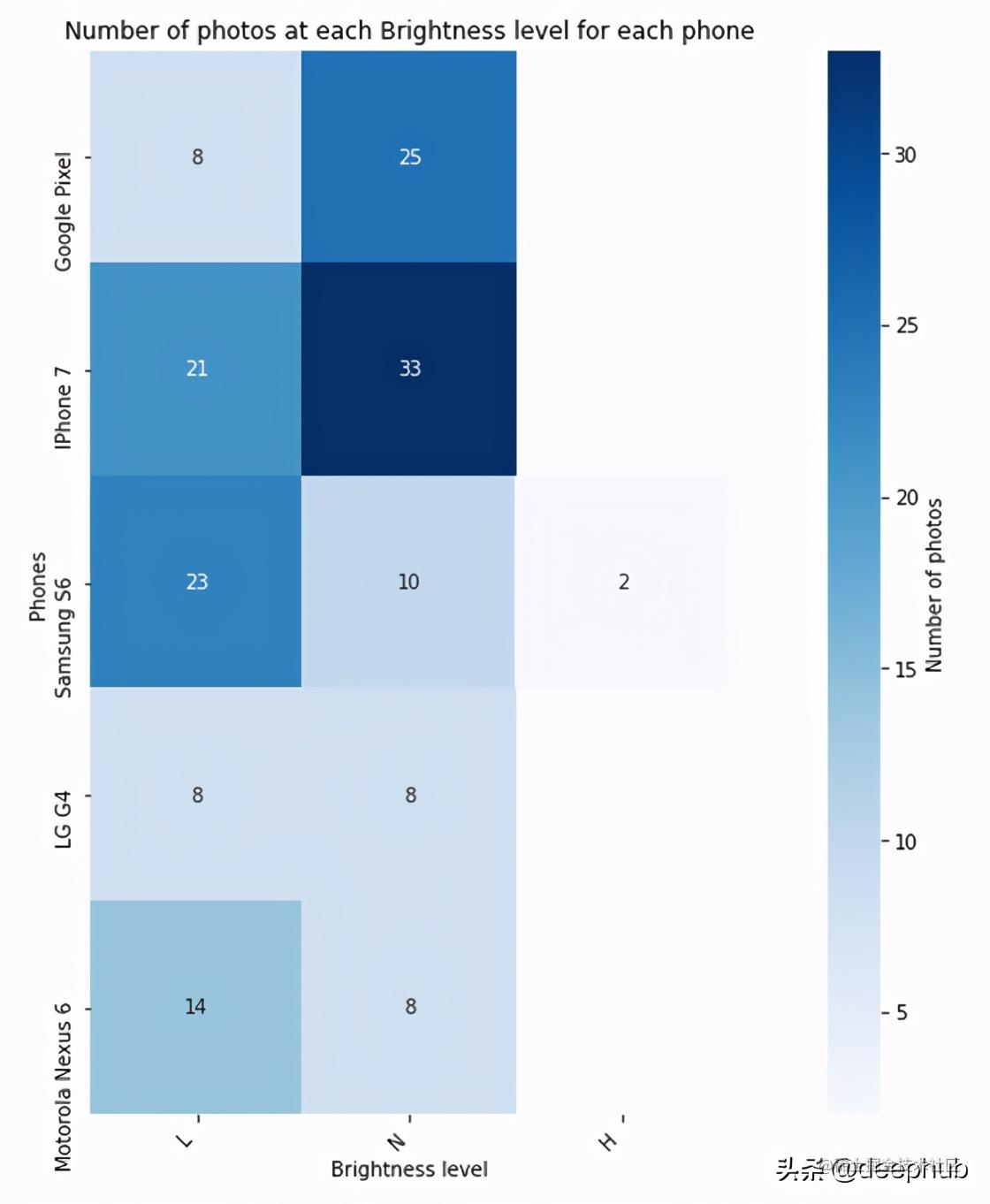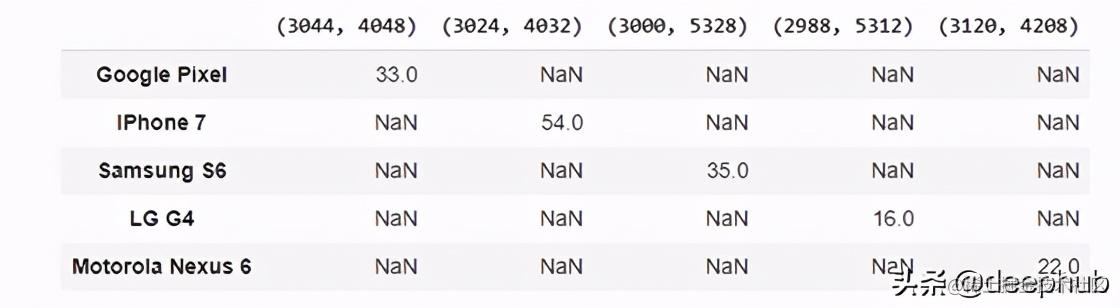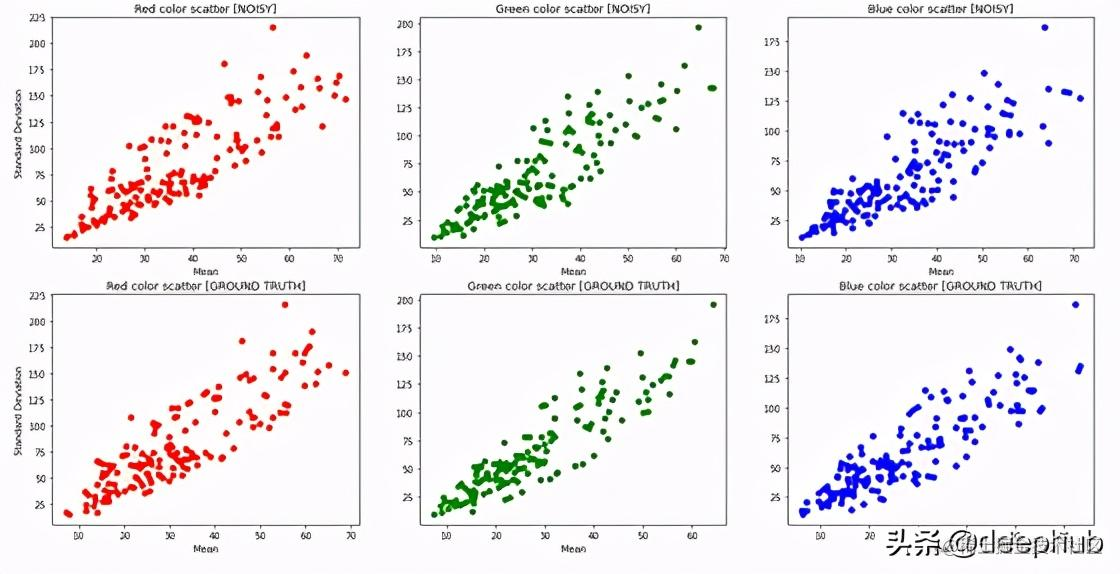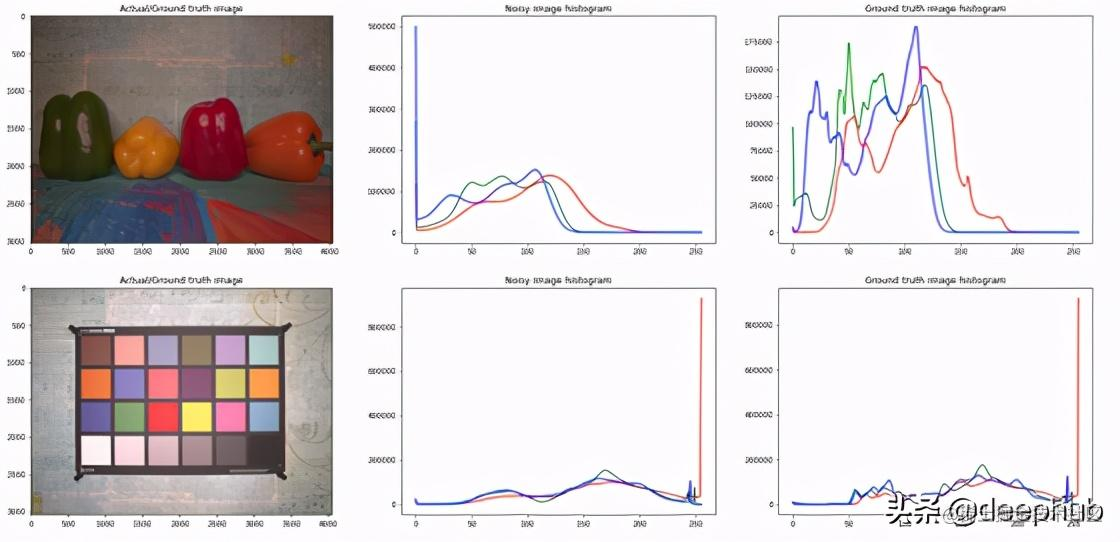传统图像去噪滤波器概述

NLM的公式，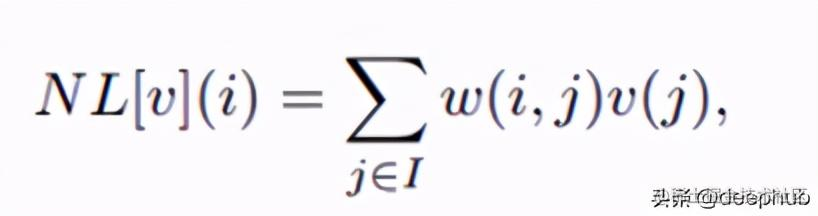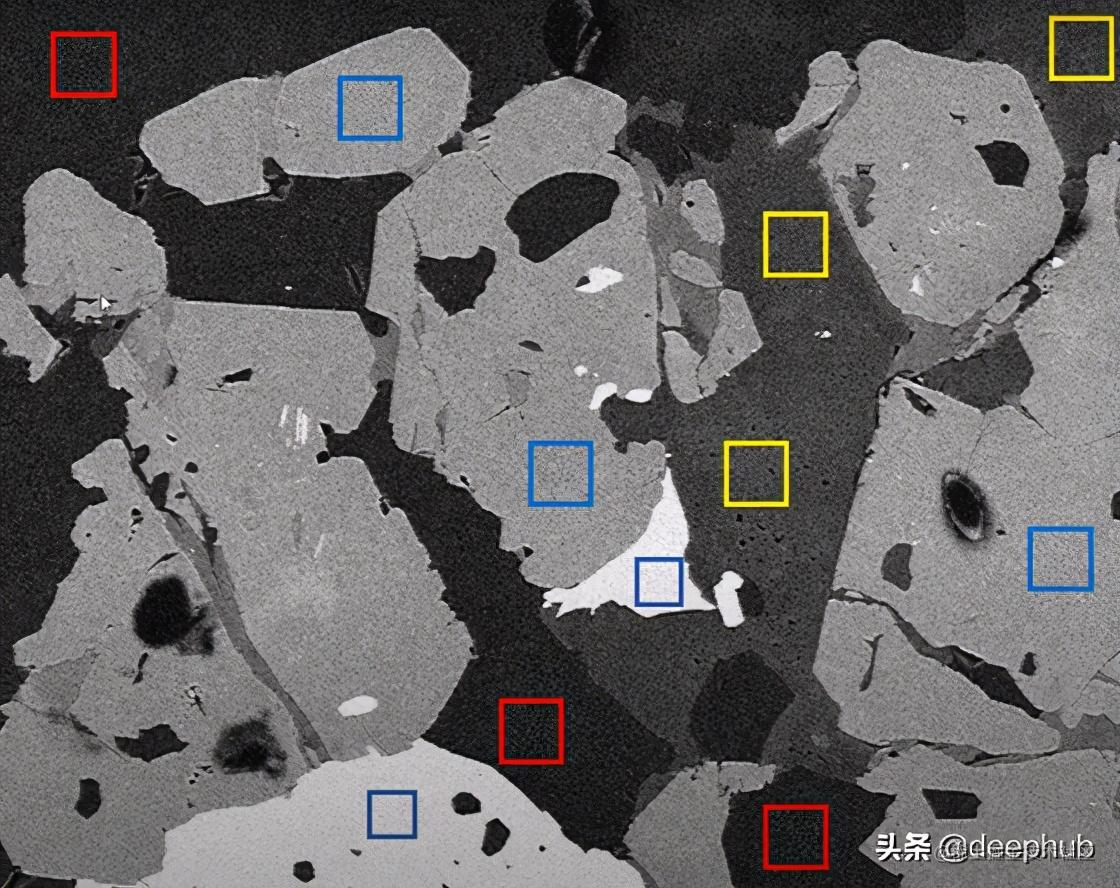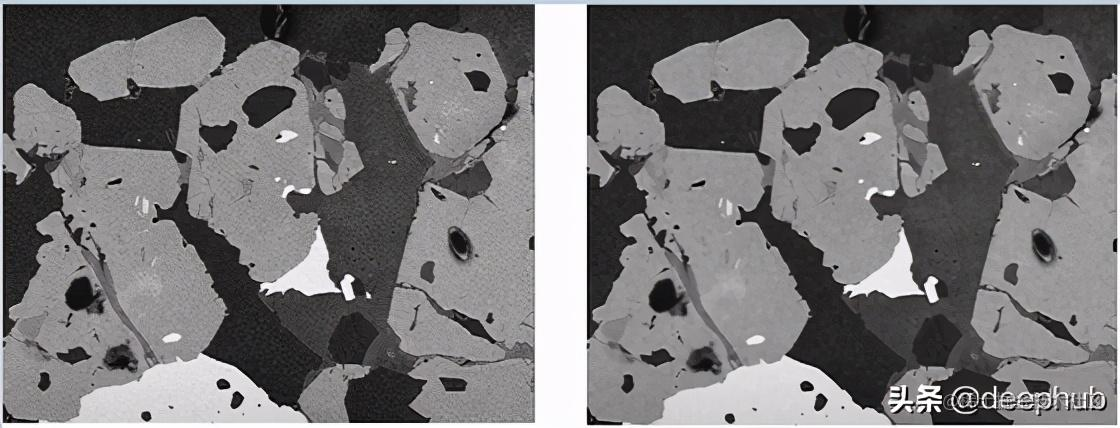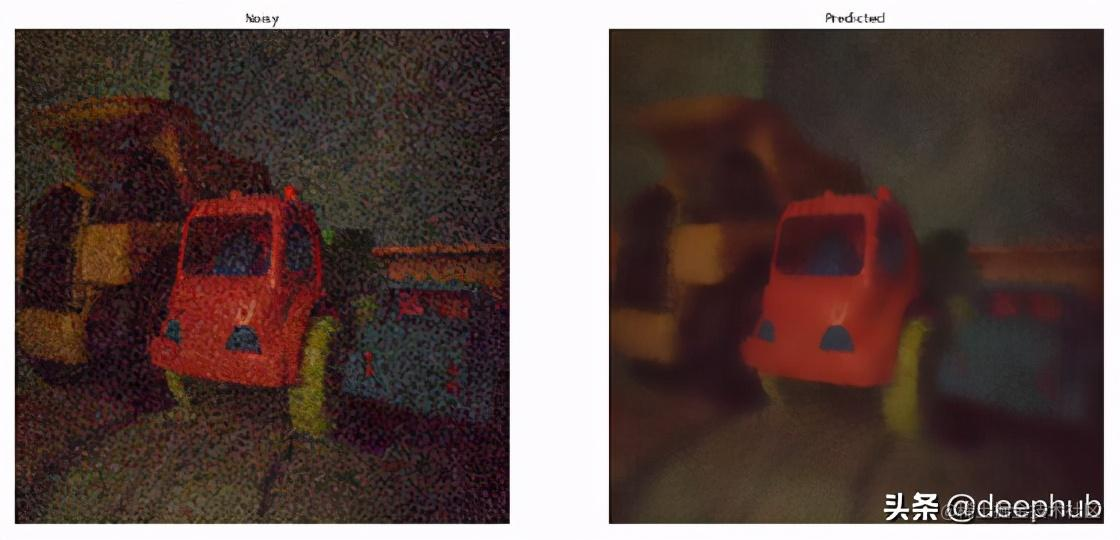用于图像去噪的深度学习模型

REDNet、MWCNN、PRIDNet

REDNet -Residual Encoder-Decoder Networks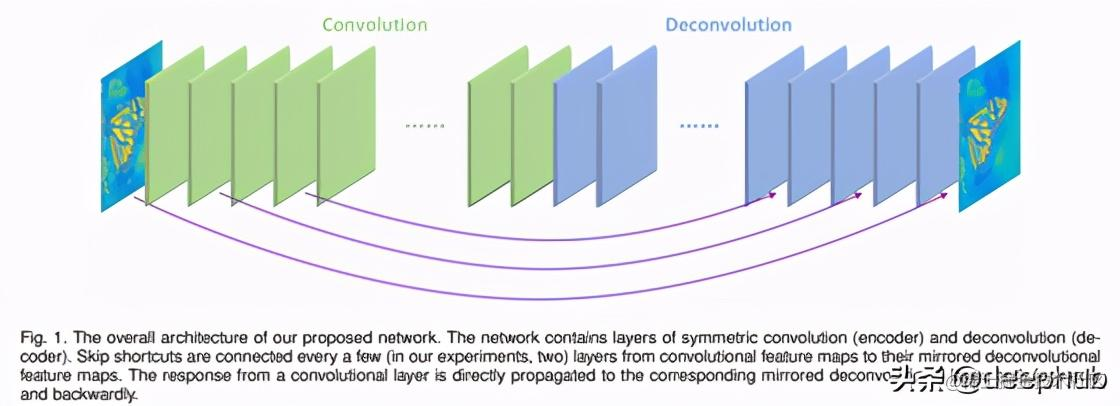input_0 = Input(shape=(256,256,3), name="input_layer")
conv_layer_1 = Conv2D(filters=256, kernel_size=2, padding='same', name="conv_1")(input_0)
conv_layer_2 = Conv2D(filters=256, kernel_size=2, padding='same', name="conv_2")(conv_layer_1)
conv_layer_3 = Conv2D(filters=256, kernel_size=3, padding='same', name="conv_3")(conv_layer_2)
conv_layer_4 = Conv2D(filters=256, kernel_size=3, padding='same', name="conv_4")(conv_layer_3)
conv_layer_5 = Conv2D(filters=128, kernel_size=3, padding='same', name="conv_5")(conv_layer_4)

deconv_layer_5 = Conv2DTranspose(filters=256, kernel_size=2, padding='same', name="deconv_5")(conv_layer_5)
deconv_layer_4 = Conv2DTranspose(filters=256, kernel_size=2, padding='same', name="deconv_4")(deconv_layer_5)
deconv_layer_3 = Conv2DTranspose(filters=256, kernel_size=3, padding='same', name="deconv_3")(deconv_layer_4)
deconv_layer_2 = Conv2DTranspose(filters=128, kernel_size=3, padding='same', name="deconv_2")(deconv_layer_3)
deconv_layer_1 = Conv2DTranspose(filters=3, kernel_size=3, padding='same', name="deconv_1")(deconv_layer_2)

model = Model(inputs=[input_0], outputs=[out])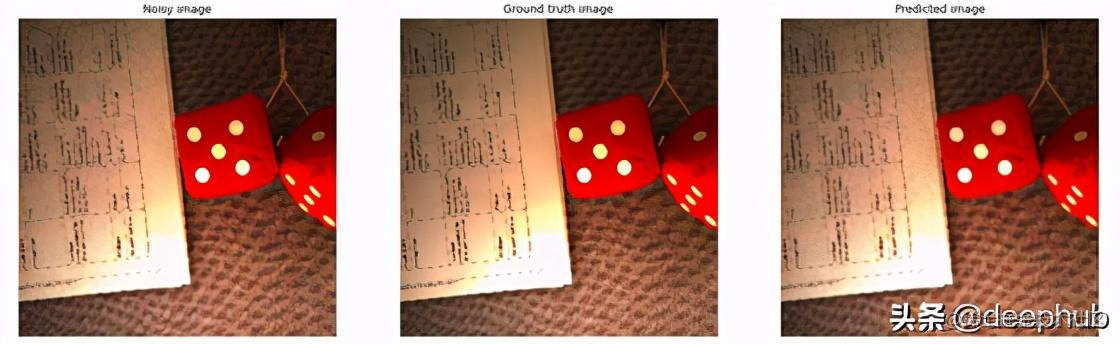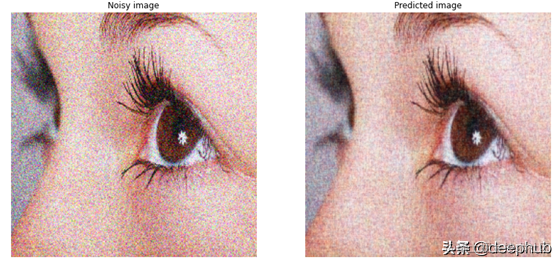MWCNN — Multi-level Wavelet CNN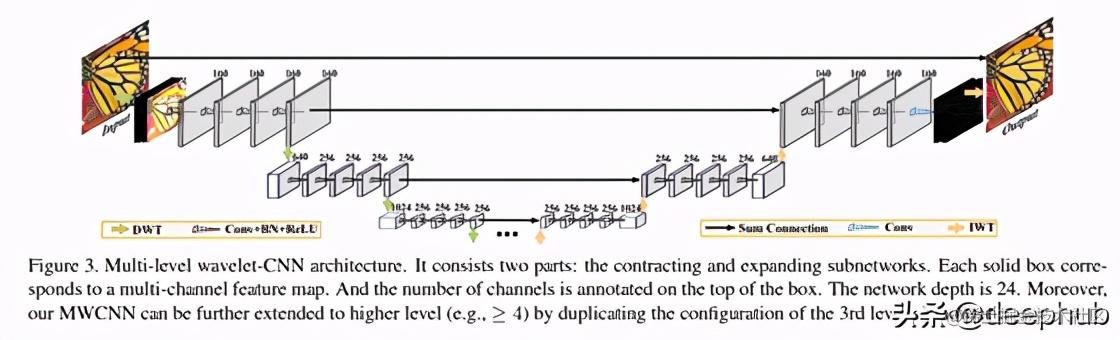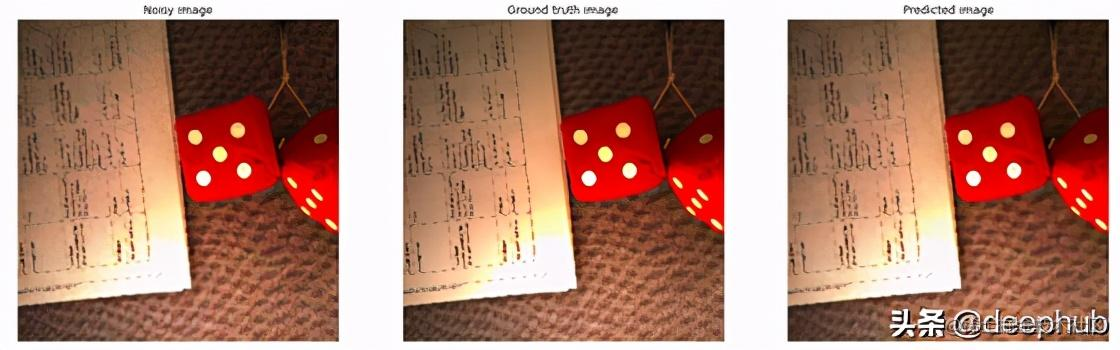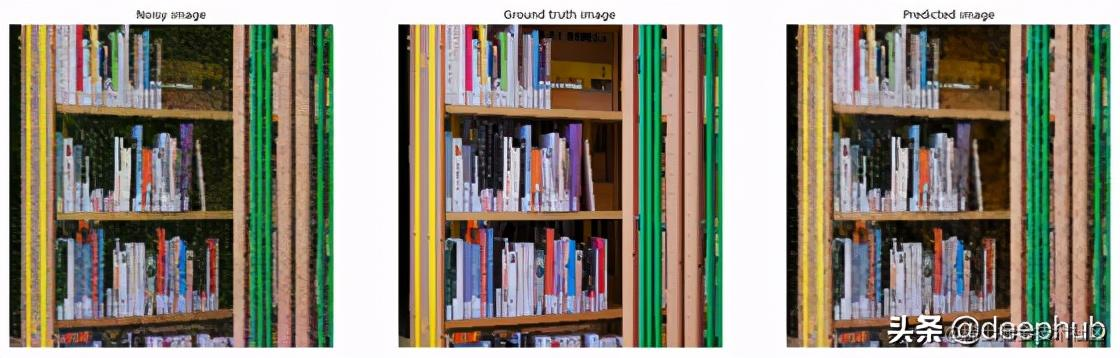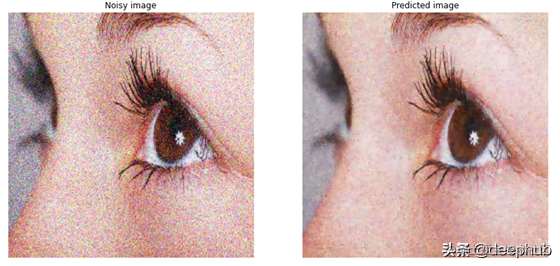PRIDNet — Pyramid Real Image Denoising Network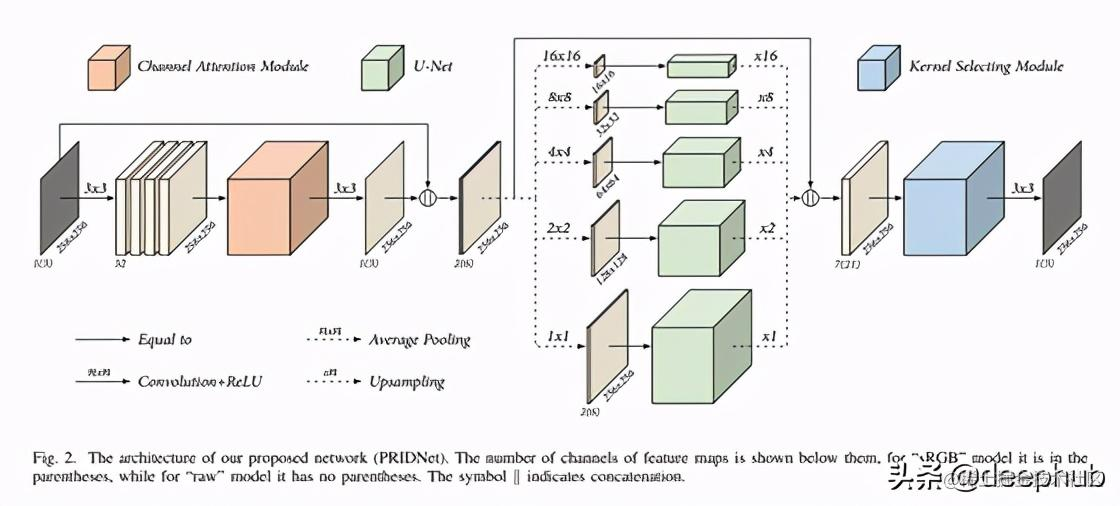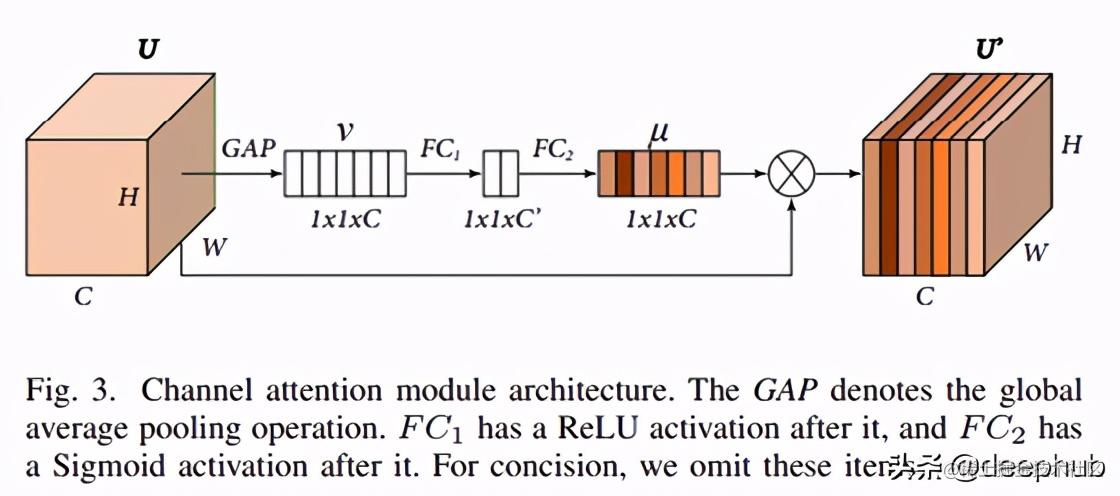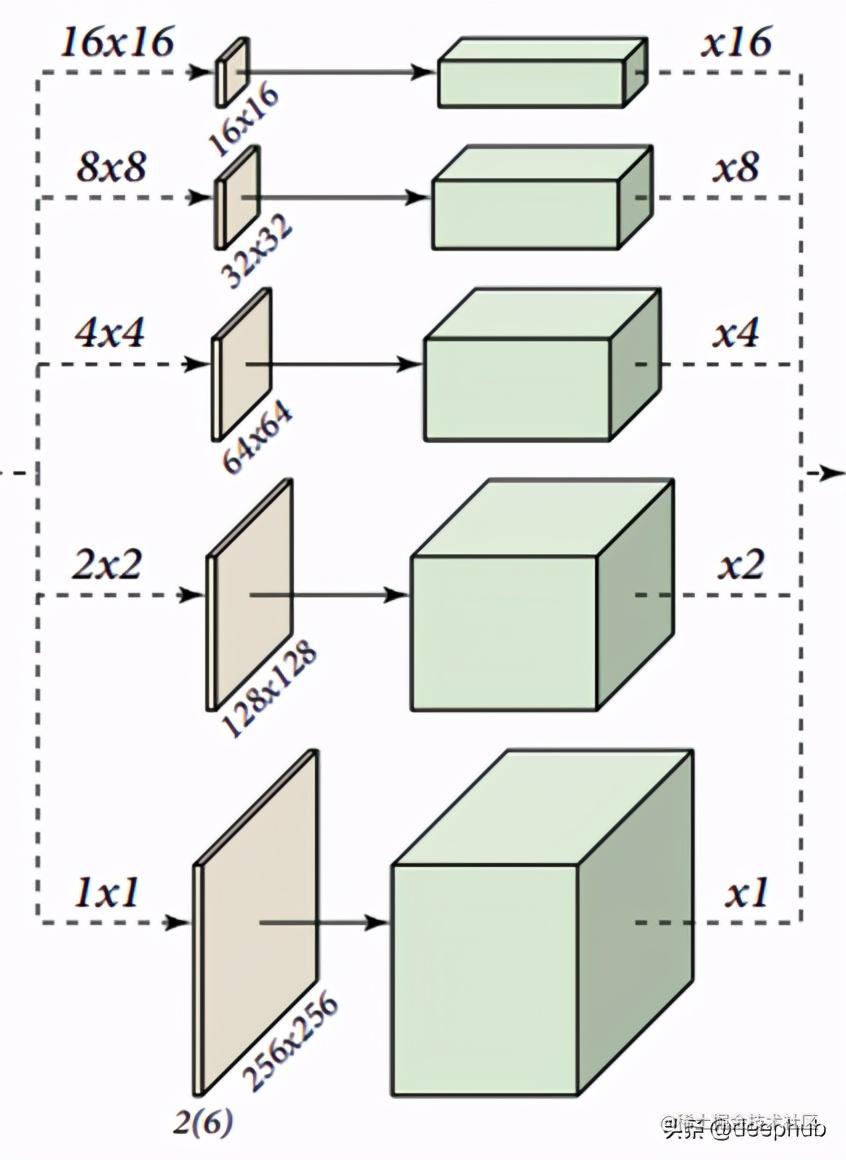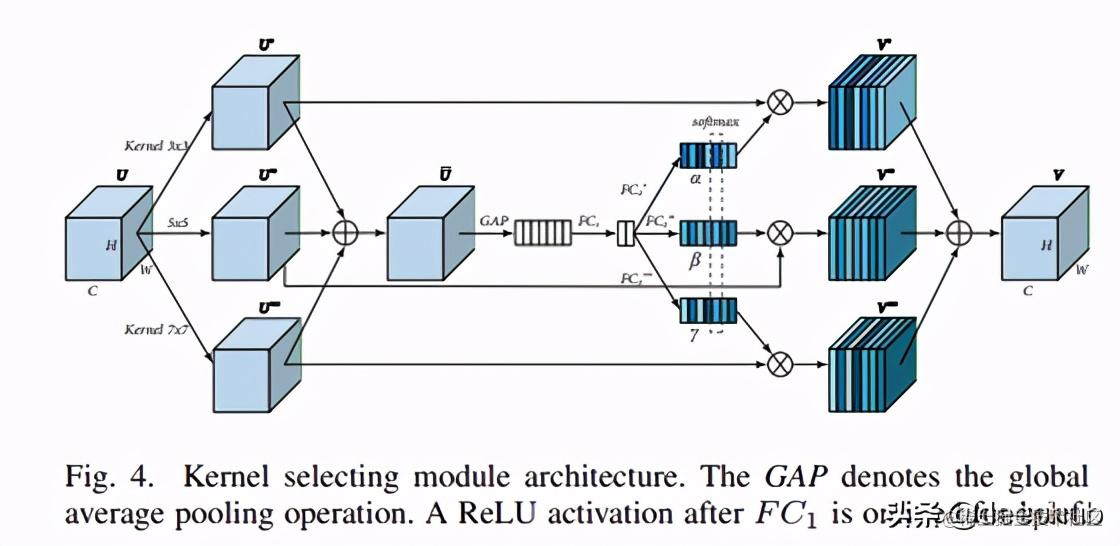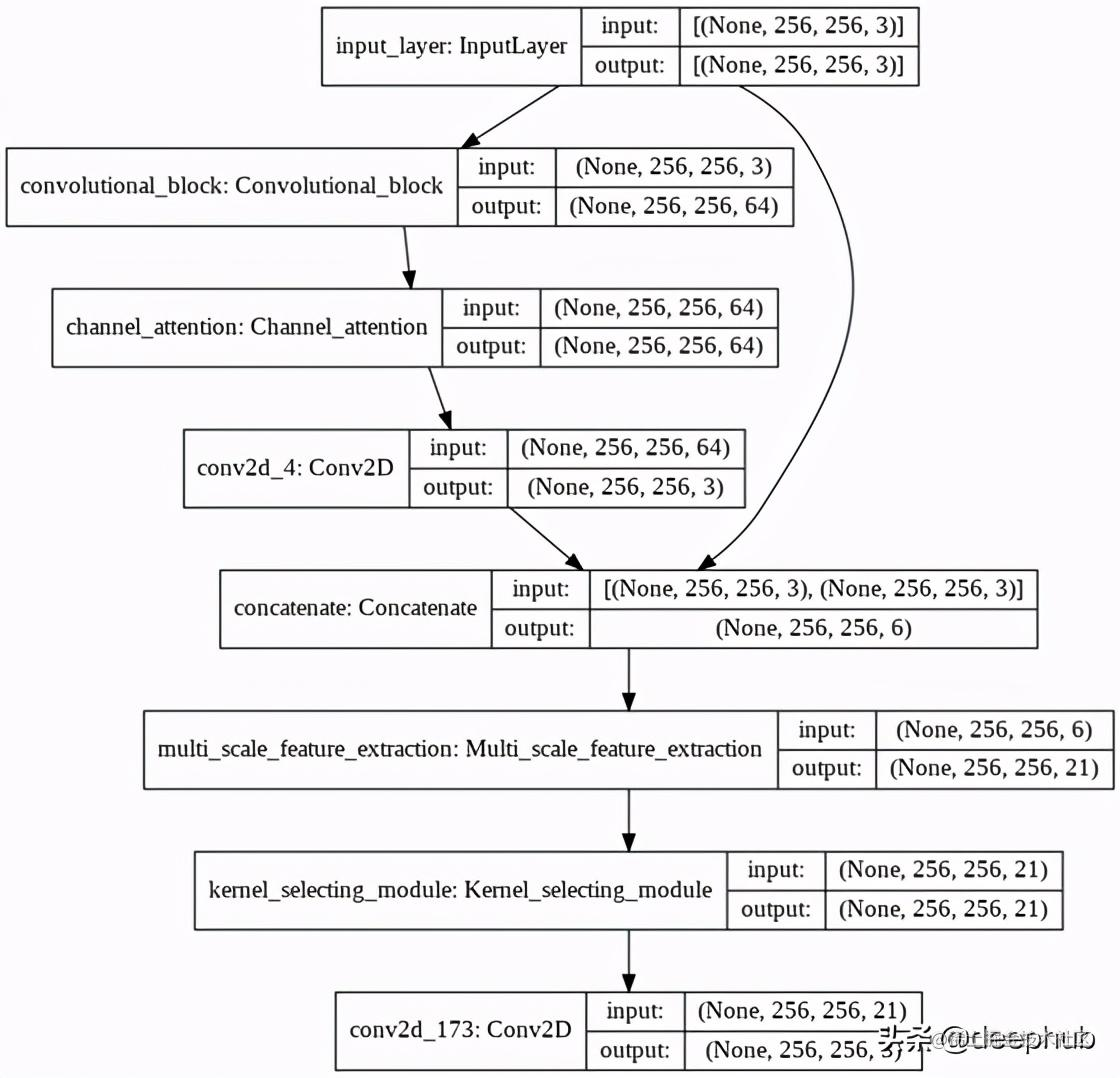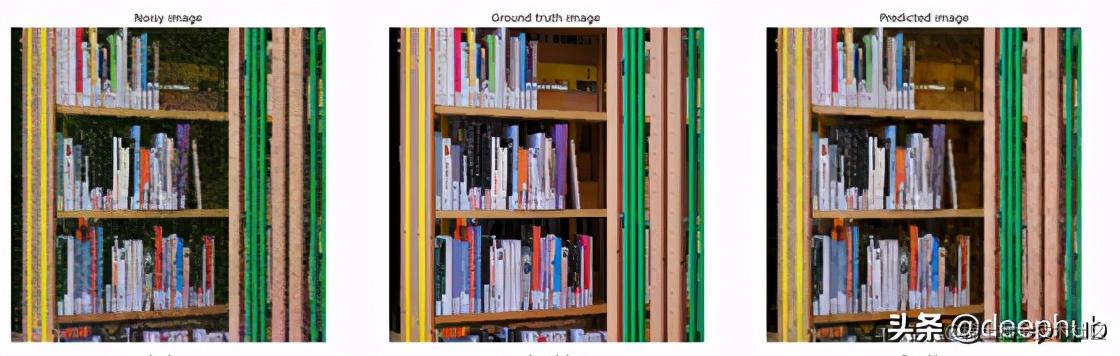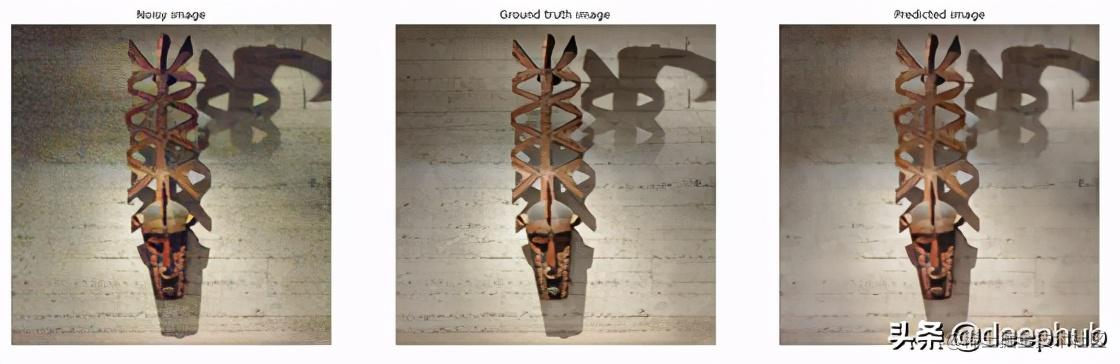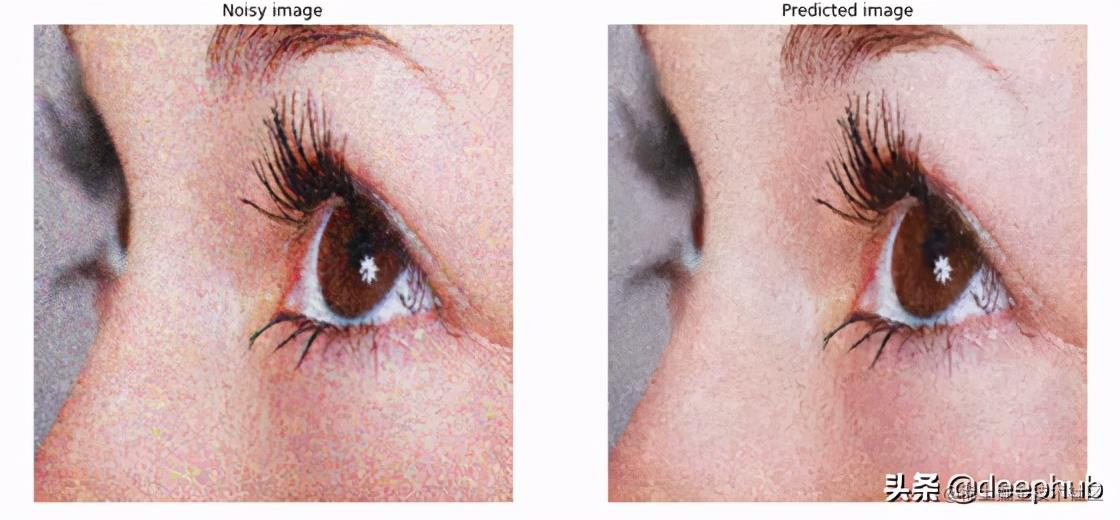结果对比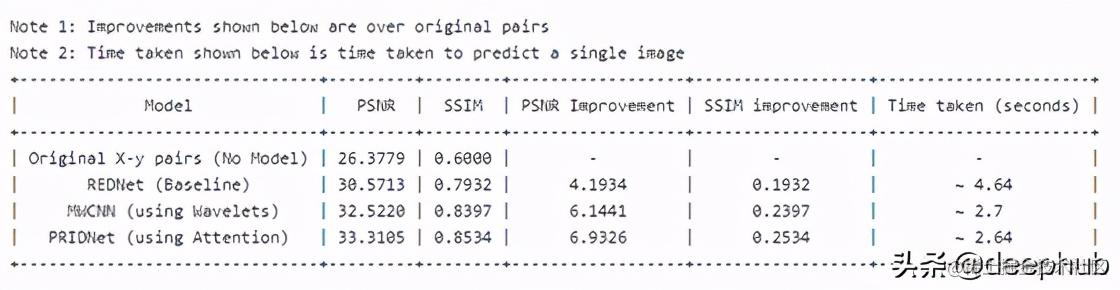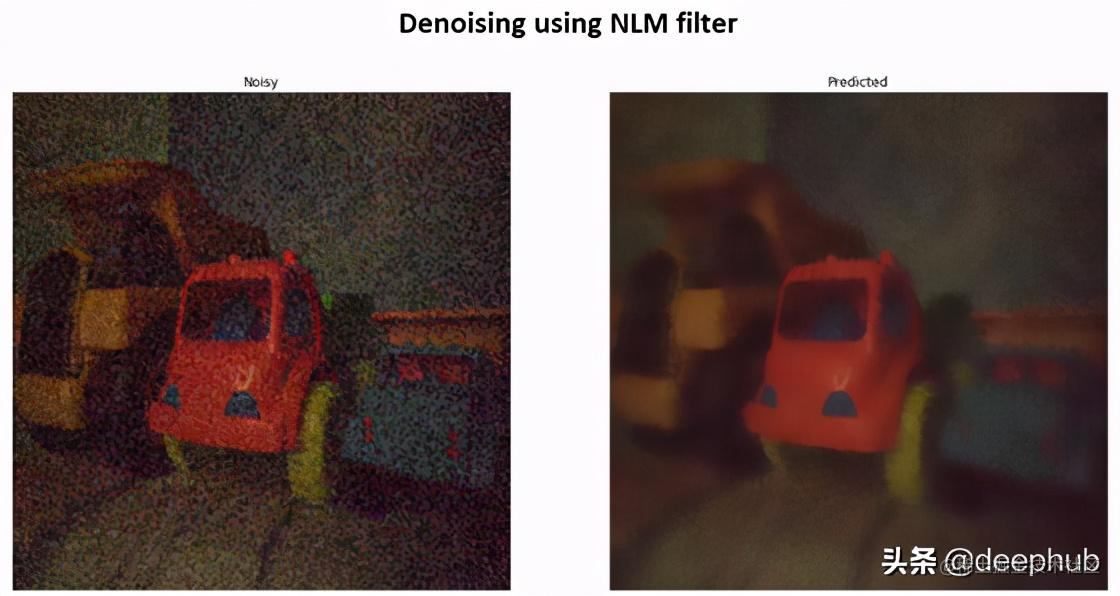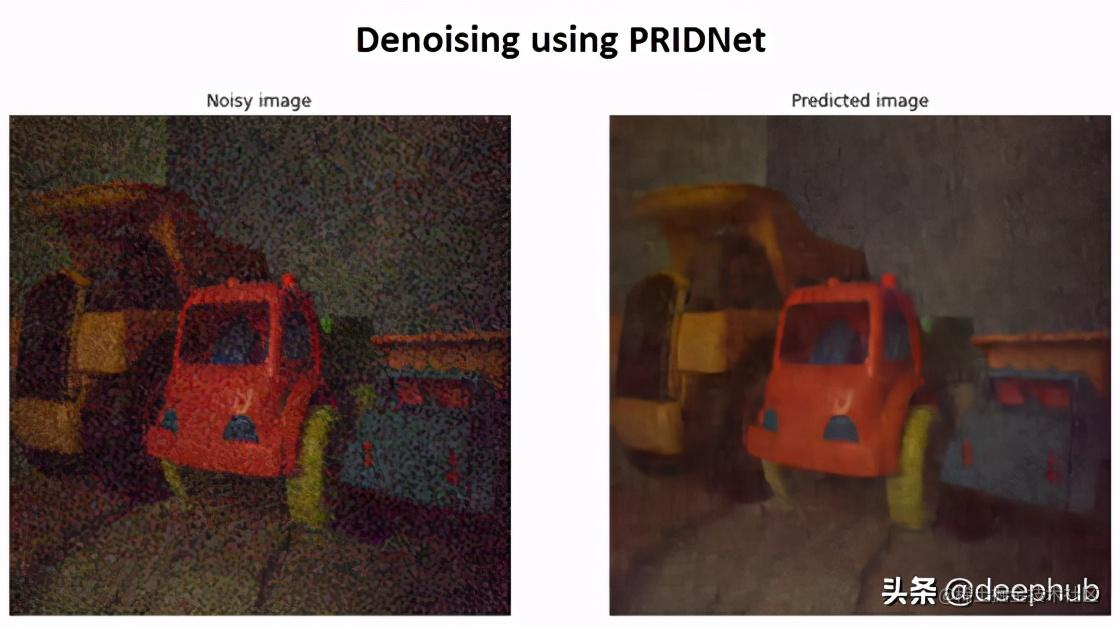• 黄色卡车的车顶区域
• 橙色卡车的座位
• 蓝色卡车中的橙色大灯
• 蓝色卡车的车顶（观察阴影）
• 地板中间的两个细条纹